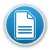I can… 4 5 6 7

## Resources:

Understand that spreadsheets, are a tool used to collect, manage, analyze and visualize data. D D D DCreating a Spreadsheet and a Graph – A Lesson for Grades 3-7

Identify and explain terms and concepts related to spreadsheets (i.e. cell, column, row, values, labels, chart, graph) E E D D

Enter/edit data and text into a spreadsheet and perform calculations using formulas E E D DCreating a Spreadsheet and a Graph – A Lesson for Grades 3-7
Use mathematical symbol and text in a spreadsheet E E D D
Utilize spreadsheet data to create tables, charts and graphs. E E D DCreating a Spreadsheet and a Graph – A Lesson for Grades 3-7

Use spreadsheets to make predictions, solve problems and draw conclusions. E E D
E = Emerging   D= Developing    P= Proficient   (O=optional) 4 5 6 7 Return to Technology Skills by Grade

Click here if you have a resource that you would like to share.